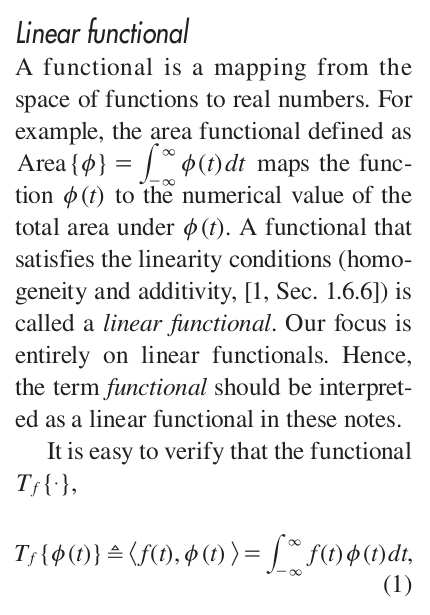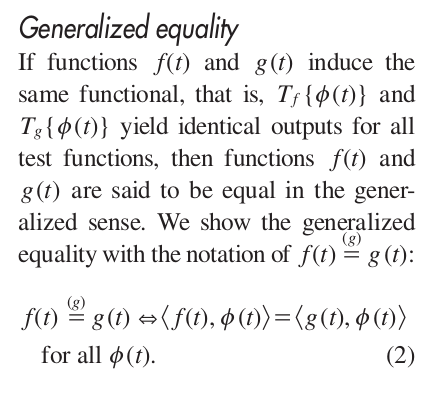# Comments on “Proper Definition and Handling of Dirac Delta Functions” by C. Candan.

An interesting paper on the true nature of the impulse function we use so much in signal processing.

The impulse function, also called the Dirac delta function, is commonly used in statistical signal processing, and on the CSP Blog (examples: representations and transforms). I think we’re a bit casual about this usage, and perhaps none of us understand impulses as well as we might.

Enter C. Candan and The Literature [R155].

Candan explains what a generalized function is, and focuses on the impulse and applications of the impulse that are useful to engineers specializing in statistical signal processing. I encourage you to go get the paper and read the whole thing, but I’m going to entice you further by discussing a few highlights.

Candan starts out by defining the linear functional, which is a function of a function. So a functional takes an entire function as input and returns a number as output if we express the idea in systems-oriented language.Figure 1. Candan in The Literature [R155] defines the linear functional, a function of a function. The function defines the functional, and so appears as a subscript on the functional, whereas the function is just any ordinary function.

The next step is to define a kind of equality using functionals: generalized equality. Two functions are equal in this generalized sense if their two functionals produce the same result when applied to all test functions$\phi(t)$ in some broad class of interest.Figure 2. The notion of generalized equality in the context of functionals.

Then Candan starts to focus on a particular set of functionals that will lead to the impulse. For example, functionals defined by a rectangle.Figure 3. Candan’s illustration of how a sequence of unit-area rectangles with decreasing widths leads to an intuitive notion of the impulse (delta) function: zero everywhere except at , where it is undefined.

Then the idea is to sneak up on the delta function by looking at a sequence of functions (the$f(t)$ and$g(t)$ that define the functional, not the test functions$\phi(t)$). For example, consider the sequence of unit-area rectangles shown in Figure 3 (Candan’s Figure 1). (See also SPTK Signal Representations Figure 3 and Eq. (23).) The limit of the sequence of rectangles doesn’t exist in the ordinary sense, since the limit function at$t=0$ is unbounded. But Candan uses that sequence to define the delta function in terms of generalized equality. A similar sequence of sinc($t$) functions, illustrated in Figure 2, also leads to the delta function.Figure 4. Illustration of how a sequence of progressively narrower sinc functions converges, in the generalized equality sense, to the impulse function.

This development leads to the various properties of the impulse function that we use in signal processing, but expressed with a notation that reminds the reader about the connection to generalized equality. Candan’s table of these familiar properties is reproduced in Figure 5.Figure 5. Useful properties of the impulse function. For signal processing in the context of communication signals, the Sifting and Convolution properties are most important.

The payoff of this unusually careful treatment of the impulse function comes when Candan looks at some familiar examples from probability theory and communication-signal modeling. In Candan’s second example, he wants to find the probability density function for a random variable that is the square of a second random variable. The sequence of equations for this example is shown in Figure 6. If you’ve ever read a probability-theory book with this example (The Literature [R149]), or tried it yourself, you’ll realize this is an easy way to solve the problem compared to conventional solutions.

The (a) equality follows from elementary probability theory–you integrate the joint density over one variable to obtain the density for the other. The (b) equality follows from the definition of conditional probability densities, and the (c) equality arises because if the random variable$X$ is known to take the value$x$, then the value of the random variable$Z$ is determined, so that the conditional density is an impulse centered at$z=x^2$. Continuing on to (d), we use the fact that the impulse is an even function. To obtain equality (e), we use the Advanced Scaling property in Figure 5 together with$x^2-z = (x+\sqrt{z})(x-\sqrt{z})$. Finally, (f) follows from the Sifting property.Figure 6. An example of the ease with which the impulse function can be used to solve problems in probability theory. Here we seek the probability density function for a random variable that is the square of a random variable with known density function .

Example 4, in Figure 7, shows how to use the generalized equality and impulse properties to show that the Fourier transform of a constant is equal to (in the generalized sense) an impulse function.